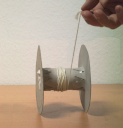#Theoretical Mechanics IPSP

Jürgen Vollmer, Universität Leipzig

### Site Tools

book:chap6:lagrange
This version (2023/06/05 20:15) was approved by jv.

# 6. Integrable DynamicsMarguerite Martyn, 1914
Figure 6.1: The point-particle idealization of a girl on a swing is the mathematical pendulum of Figures 1.2 and 1.3.

In Chapter 5 we considered objects that consist of a mass points with fixed relative positions, like a flying and spinning ping-pong ball. Rather than providing a description of each individual mass element, we established equations of motion for their center of mass and the orientation of the body in space. From the perspective of theoretical mechanics the fixing of relative positions is a constraint to their motion, just as the ropes of a swing enforces a motion on a one-dimensional circular track, rather than in two dimensions. The deflection angle θ of the pendulum, and the center of mass and orientation of the ball are examples of generalized coordinates that automatically take into account the constraints.

In this chapter we discuss how to set up generalized coordinates and how to find the associated equations of motion. The discussion will be driven by examples. The examples will be derived from the realm of integrable dynamics. These are systems where conservation laws can be used to break down the dynamics into separate problems that can be interpreted as motion with a single degree of freedom.

At the end of the chapter you know why coins run away rolling on their edge, and how the speed of a steam engine was controlled by a mechanical device. Systems where the dynamics is not integrable will subsequently be addressed in Chapter 7.

The PDF file of the chapter is available here.

I am curious to see your questions, remarks and suggestions: